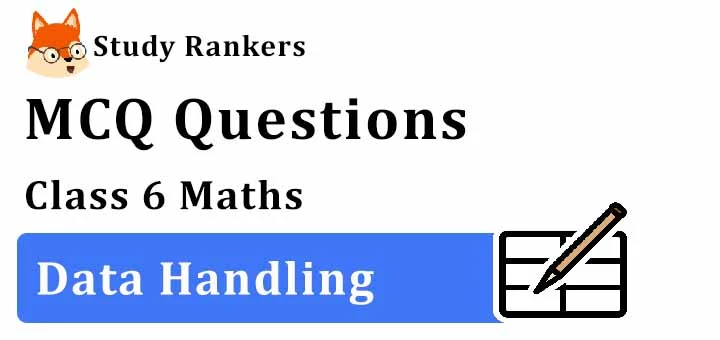## MCQ Questions for Class 6 Maths: Ch 9 Data Handling1. If O represents 5 eggs how many eggs does OOOO represent?
(a) 4
(b) 16
(c) 20
(d) 25
► (c) 20

2. The ________ of each bar gives the required information.
(b) height
(c) length
(d) None of these
► (c) length

3. What is a graph drawn using vertical bars called?
(a) A bar graph
(b) A line graph
(c) A pictograph
(d) A pie graph
► (a) A bar graph

4. Which of the following is the probability of an impossible event?
(a) 0
(b) 1
(c) 2
(d) None of these
► (a) 0

5. A symbol is used to represent 100 flowers. How many symbols are to be drawn to show 800 flowers?
(a) 8
(b) 12
(c) 10
(d) 80
► (a) 8

6. If  ✱ represents 5 balloons, number of symbols to be drawn to represent 60 balloons is
(a) 5
(b) 60
(c) 10
(d) 12
► (d) 12

7. A coin is tossed. Which of the following is the probability of getting a head or tail?
(a) 0
(b) 1
(c) 1/2
(d) None of these
► (c) 1/2

8. Bars of uniform width can be drawn ____________ with equal spacing between them and then the length of each bar represents the given number.
(a) horizontally
(b) horizontally or vertically
(c) vertically
(d) None of these
► (b) horizontally or vertically

9. What is the number of houses represented by the figure 个个个个  if each symbol represents 15 houses?
(a) 60
(b) 20
(c) 15
(d) 40
► (a) 60

10. If ⬜⬜⬜⬜ stands for 300, what doc ⬜⬜ stand for?
(a) 60
(b) 100
(c) 120
(d) 260
► (b) 100

11. A _____ is a collection of numbers gathered to give some information.
(a) frequency
(b) data
(c) tally mark
(d) None of these
► (b) data

12. A ________ represents data through pictures of objects.
(a) histogram
(b) pictograph
(c) bar graph
(d) None of these
► (b) pictograph